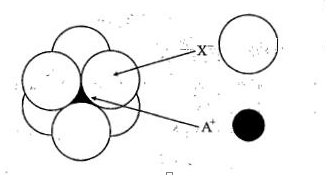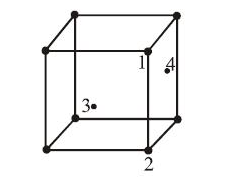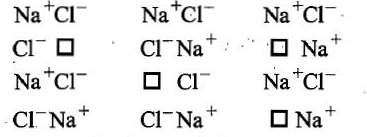Lithium borohydride (LiBH4), crystallises in an orthorhombic system with 4 molecules per unit cell. The unit cell dimensions are:- a = 6.81 Å , b = 4.43 Å ,c = 7.17 Å. If the molar mass of LiBH4 is
21.76 g mol-1.The density of the crystal is:-

1. 0.668 gcm-3
2. 0.585 gcm2
3. 1.23 gcm-3

4. None of these

Concept Questions :-

Density/Formula/packing fraction/ Semiconductors
High Yielding Test Series + Question Bank - NEET 2020

Difficulty Level:

The arrangement of Xions around A+ ion in solid AX is given in the figure (not drawn to scale).
If the radius of X is 250 pm,the radius of A+ is:(a)104 pm
(b)125 pm
(c)183 pm
(d) 57 pm

Concept Questions :-

High Yielding Test Series + Question Bank - NEET 2020

Difficulty Level:

In an f.c.c. unit cell. atoms are numbered as shown below.The atoms not touching each other are (Atom numbered 3 is face centre of front face).(A) 3&4
(B) 1&3
(C) 1&2
(D) 2&4

Concept Questions :-

Introduction and Type of Crystal System
High Yielding Test Series + Question Bank - NEET 2020

Difficulty Level:

A solid has a bcc structure. If the distance of closest approach between the two atoms is 1.73Å . The edge length of the cell is:

(a) 200pm

(b) $\sqrt{3}}{\sqrt{2}}$ pm

(c) 142.2 pm

(d) $\sqrt{2}$pm

Concept Questions :-

Density/Formula/packing fraction/ Semiconductors
High Yielding Test Series + Question Bank - NEET 2020

Difficulty Level:

Which is covalent solid?

(a) Fe2O3

(b) Diamond

(c) Graphite

(d) All of these

Concept Questions :-

Introduction and Type of Crystal System
High Yielding Test Series + Question Bank - NEET 2020

Difficulty Level:

What type of crystal defects is indicated in the diagram given below?(a) Frenkel and Schottky defects
(b) Schottky defect
(c) Interstitial defect
(d) Frenkel defect

Concept Questions :-

Imperfections in Solids
High Yielding Test Series + Question Bank - NEET 2020

Difficulty Level:

The anions (A) form hexagonal closest packing and atoms (C) occupy only 2/3 of octahedral voids in
it. then the general formula of the compound is-

(A) CA

(B) A2

(C) C2A3

(D) C3A2

Concept Questions :-

Density/Formula/packing fraction/ Semiconductors
High Yielding Test Series + Question Bank - NEET 2020

Difficulty Level:

The mass of a unit cell of CsCl corresponds to:-

1. 8Cs+ and Cl-

2. 1Cs+ and 6Cl-

3. 1Cs+ and 1Cl-

4. 4Cs+ and Cl-

Concept Questions :-

Introduction and Type of Crystal System
High Yielding Test Series + Question Bank - NEET 2020

Difficulty Level:

Graphite in an example of-

(A) Ionic solid

(B) Covalent solid

(C) Vander waal's crystal

(D) Metallic crystal

Concept Questions :-

Introduction and Type of Crystal System
High Yielding Test Series + Question Bank - NEET 2020

Difficulty Level:

Which arrangement of electrons leads to anti-ferromagnetism?
(a)  ↑↑↑↑
(b)  ↑↓↑↓
(c) Both (a) and (b)
(d) None of these

Concept Questions :-

Magnetic Behaviour of an Element/Compound